# AP Statistics Multiple-Choice Practice Questions: Summarizing Distributions 3

### Test Information12 questions28 minutes

1. Suppose 10% of a data set lie between 40 and 60. If 5 is first added to each value in the set and then each result is doubled, which of the following is true?

2. The 70 highest dams in the world have an average height of 206 meters with a standard deviation of 35 meters. The Hoover and Grand Coulee dams have heights of 221 and 168 meters, respectively. The Russian dams, the Nurek and Charvak, have heights with z-scores of +2.69 and -1.13, respectively. List the dams in order of ascending size.

3. The first 115 Kentucky Derby winners by color of horse were as follows: roan, 1; gray, 4; chestnut, 36; bay, 53; dark bay, 17; and black, 4. (You should "bet on the bay!") Which of the following visual displays is most appropriate?

4. For Question below considers the following: The graph below shows cumulative proportions plotted against grade point averages for a large public high school.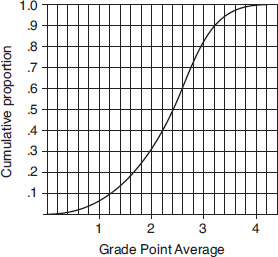What is the median grade point average?

5. For Question below considers the following: The graph below shows cumulative proportions plotted against grade point averages for a large public high school.What is the interquartile range?

6. The following dotplot shows the speeds (in mph) of 100 fastballs thrown by a major league pitcher.Which of the following is the best estimate of the standard deviation of these speeds?

7.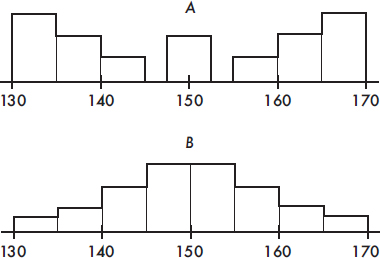Which of the following statements about the two histograms above is true?

8. Consider the following back-to-back stemplots comparing car battery lives (in months) of samples of two popular brands.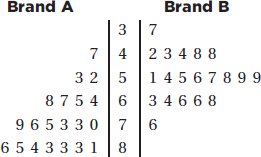Which of the following are true statements?

I.The sample sizes are the same.

II.The ranges are the same.

III.The variances are the same.

IV.The means are the same.

V.The medians are the same.

9. The following boxplots were constructed from SAT math scores of boys and girls at a high school: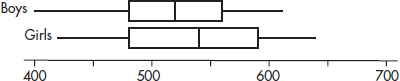Which of the following is a possible boxplot for the combined scores of all the students?

10. Question below refers to the following population pyramids (source: U.S. Census Bureau).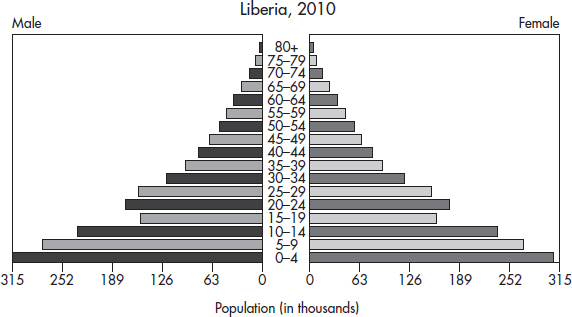What is the approximate median age of the Liberian population?

11. Question below refers to the following population pyramids (source: U.S. Census Bureau).Which country has more children younger than 10 years of age?

12. Question below refers to the following population pyramids (source: U.S. Census Bureau).Which of the following statements are plausible, given the graphs?

I.Canadian women tend to live longer than men.

II.The recent civil war in Liberia, with the extensive use of child soldiers, has had an impact on the population age distribution.

III.Canadian demographics show a decreasing birth rate.